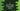# C program to print all uppercase and lowercase letters in the alphabet### Write a C program to print all Uppercase and Lowercase letters in the alphabet:

In this example program, we will learn how to print the uppercase and lowercase letters of the alphabet in C programming language. We will use one for loop to print the characters. Let’s take a look into the program :

### Method 1: C program with `for` loop to print the uppercase and lowercase letters:

The following program uses two `for` loops to print the uppercase and lowercase letters of the alphabet:

``````#include <stdio.h>

int main()
{
//1
char ch;

//2
printf("Uppercase characters:\n");
//3
for (ch = 'A'; ch <= 'Z'; ch++)
{
printf("%c ", ch);
}

//4
printf("\nLowercase characters:\n");
for (ch = 'a'; ch <= 'z'; ch++)
{
printf("%c ", ch);
}

return 0;
}``````

#### Explanation :

The commented numbers in the above program denote the step number below:

1. Create one character variable `ch`.
2. Start printing the uppercase characters.
3. Start a `for` loop. The loop runs from `ch = 'A'` to `ch = 'Z'`. Inside the loop, we are printing the value of `ch`. It increases the value of `ch` by `1` on each iteration i.e. the ASCII value of the character defined by the variable `ch` is increased by 1.
4. The second `for` loop is printing the lowercase characters. It runs from `ch = 'a'` to `ch = 'z'`.

#### Sample output of the program :

If you run the above program, it will print the below output:

``````Uppercase characters :
A B C D E F G H I J K L M N O P Q R S T U V W X Y Z
Lowercase characters :
a b c d e f g h i j k l m n o p q r s t u v w x y z``````

### Method 2: C program with `while` loop to print the uppercase and lowercase letters:

We can also use two `while` loops to print the uppercase and lowercase characters of the alphabet. The following program shows how to print the uppercase and lowercase letters with two `while` loops:

``````#include <stdio.h>

int main()
{
char ch = 'A';

printf("Uppercase characters:\n");

while (ch <= 'Z')
{
printf("%c ", ch);
ch++;
}

ch = 'a';
printf("\nLowercase characters:\n");
while (ch <= 'z')
{
printf("%c ", ch);
ch++;
}

return 0;
}``````

Here, the `ch` variable is initialized before the loop starts and at the end of the loop, we are incrementing the value of `ch` by 1. It will print the same output.

``````Uppercase characters:
A B C D E F G H I J K L M N O P Q R S T U V W X Y Z
Lowercase characters:
a b c d e f g h i j k l m n o p q r s t u v w x y z``````

### Method 3: How to use it with `do...while` loop:

We can also write this program with `do...while` loops. The `do...while` loop is similar to a `while` loop. The only difference is that it runs the code in the `do` block and then checks the `while` condition. The following program shows how it works:

``````#include <stdio.h>

int main()
{
char ch = 'A';

printf("Uppercase characters:\n");

do
{
printf("%c ", ch);
ch++;
} while (ch <= 'Z');

ch = 'a';
printf("\nLowercase characters:\n");
do
{
printf("%c ", ch);
ch++;
} while (ch <= 'z');

return 0;
}``````